# How to Add/Remove Chart Border in Excel

When creating a chart for existing table, light grey border will be displayed around chart by default. If we want to remove chart border or add it back again (or change color), we can through editing Format Chart Area->Border Color to add or remove chart border properly. This article will show you the details about this function.

Precondition:

Prepare a table first.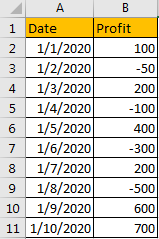Then create a chart for this table via Insert tab->Recommended Charts (select a chart).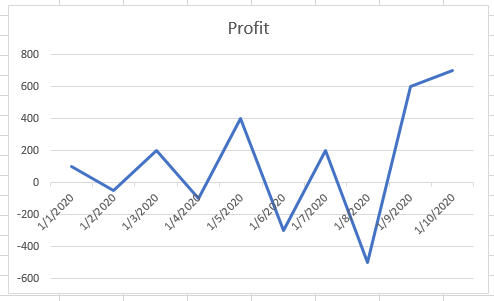Verify that border is displayed in light grey.

## Method 1: Remove Chart Border in Excel

Step 1: Click on the border, right click to load menu, select ‘Format Chart Area’.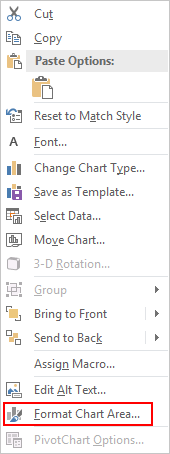Step 2: On Format Chart Area, under Chart Options click Fill & Line icon, under Border options check on No line.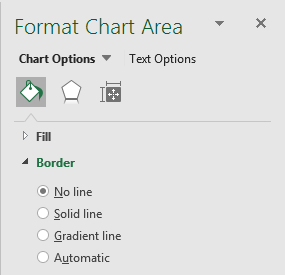Verify that now chart border is removed.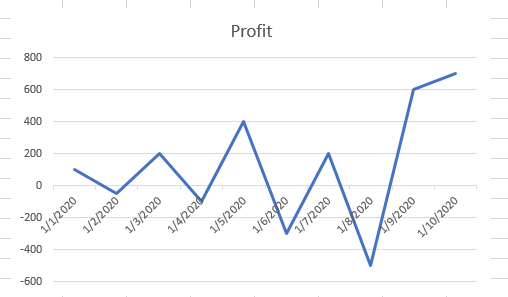## Method 2: Add Chart Border in Excel

If we want to add border back, we just need to check on Solid line, Gradient line or Automatic line per our demand.

Step 1: Still in Format Chart Area, under Fill & Line->Border, check on Solid line. Select color, you can also edit or adjust other parameters like transparency or width.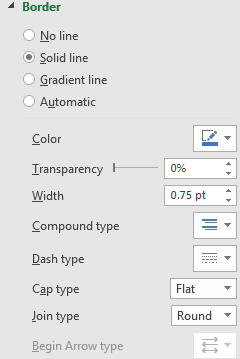Then solid border is displayed.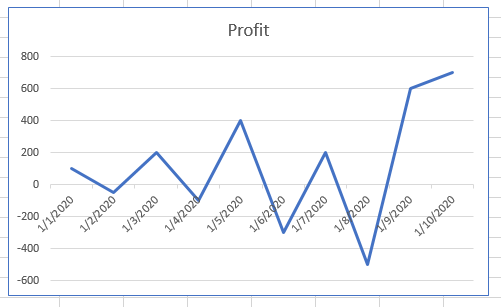Step 2: Check on Gradient line.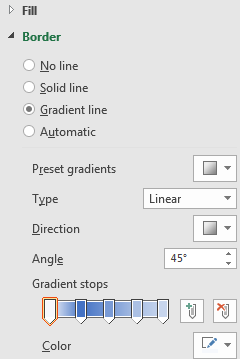Border color is displayed in gradient.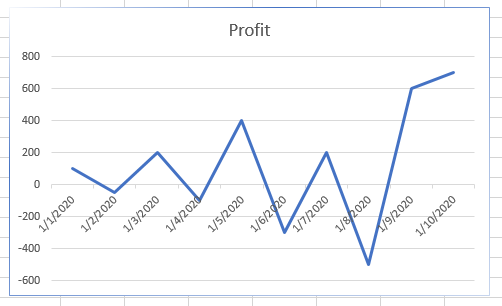Step 3: Check on Automatic line. You can get light grey border by default as the chart is created. You can also edit parameters to show different border by checking on Automatic line.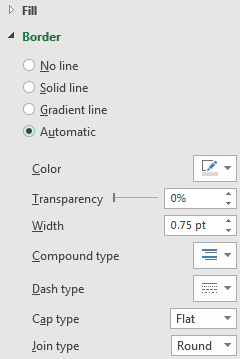Related Posts

Cap percentage values between 0 and 100

This article will talk about how to cap the percentage values between 0% and 100% in Microsoft Excel Spreadsheet or Google Sheets. If you are a newbie on Excel or google Sheets, you may be able to do this by ...

Calculate Cap Percentages to Specific Value

This article will talk about how to limit the cap percentage of a given amount to a given value in Microsoft Excel Spreadsheet or Google Sheets. If you are a newbie on Excel or google Sheets, you may be able ...

Calculate Win Loss Tie

Suppose you got a task to calculate the win, loss, and tie totals; what would you do? If you are new to Ms Excel and don't have enough experience with it, then you might do this task manually but let ...

Calculate Years Between Dates In Ms Excel

If you are an avid Ms Excel user, then you might have come across a task in which you needed to calculate the years between the dates; you might take it easy and do this task manually, which is also ...

Calculate Number of Hours between Two Times

Calculating the difference between two times might be a valuable statistic for subsequent computations or averages, whether you're producing a time sheet for staff or recording personal exercises. While Excel has a plethora of complex functions, including date and time ...

Calculate Loan Interest in Given Year

When you borrow money, you are supposed to repay it gradually. Lenders, on the other hand, want to be compensated for their services and the risk they incur by lending you money. That is, you will not just repay the ...

Calculate Interest Rate for Loan

The interest rate is the fee charged by a lender to a borrower and is expressed as a percentage of the principal—the lent amount. The interest rate on a loan is often expressed as an annual percentage rate, abbreviated as ...

Calculate Interest for Given Period

Using the IPMT function in Excel, we can compute the interest payment on any loan. This step-by-step tutorial will guide Excel users of all skill levels through the process to calculate interest for given period. Finally, the formula: =IPMT(B3/12,1,B5,-B2) The ...

How To Use Excel GCD Function

This post will guide you how to use Excel GCD function with syntax and examples in Microsoft excel. Description The Excel GCD function Returns the greatest common divisor of two or more integers. So you can use the GCD function ...

Calculate A Ratio From Two Numbers In Excel

In elementary mathematics, a ratio is a connection or comparison between two or more integers. For example, ratios are often expressed as ":" to demonstrate the relationship between two numbers. You would think that manually calculating a ratio from two ...

Sidebar